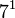# Groups of order 84

## Contents

See pages on algebraic structures of order 84| See pages on groups of a particular order

## Statistics at a glance

The number 84 has the prime factorization:$\! 84 = 2^2 \cdot 3^1 \cdot 7^1 = 4 \cdot 3 \cdot 7$

All groups of this order are solvable groups.

Quantity Value Explanation
Total number of groups up to isomorphism 15
Number of abelian groups (i.e., finite abelian groups) up to isomorphism 2 (number of abelian groups of order$2^2$) times (number of abelian groups of order$3^1$) times (number of abelian groups of order$7^1$) = (number of unordered integer partitions of 2) times (number of unordered integer partitions of 1) times (number of unordered integer partitions of 1) =$2 \times 1 \times 1 = 2$. See classification of finite abelian groups and structure theorem for finitely generated abelian groups.
Number of nilpotent groups (i.e., finite nilpotent groups) up to isomorphism 2 (number of groups of order 4) times (number of groups of order 3) times (number of groups of order 7) =$2 \times 1 \times 1 = 2$.
Number of supersolvable groups (i.e., finite supersolvable groups) up to isomorphism 13
Number of solvable groups (i.e., finite solvable groups) up to isomorphism 15 All groups of this order are solvable.
Number of simple groups up to isomorphism 0 Follows from all groups of this order being solvable.

## GAP implementation

The order 84 is part of GAP's SmallGroup library. Hence, any group of order 84 can be constructed using the SmallGroup function by specifying its group ID. Also, IdGroup is available, so the group ID of any group of this order can be queried.

Further, the collection of all groups of order 84 can be accessed as a list using GAP's AllSmallGroups function.

Here is GAP's summary information about how it stores groups of this order, accessed using GAP's SmallGroupsInformation function:

gap> SmallGroupsInformation(84);

There are 15 groups of order 84.
They are sorted by their Frattini factors.
1 has Frattini factor [ 42, 1 ].
2 has Frattini factor [ 42, 2 ].
3 has Frattini factor [ 42, 3 ].
4 has Frattini factor [ 42, 4 ].
5 has Frattini factor [ 42, 5 ].
6 has Frattini factor [ 42, 6 ].
7 - 15 have trivial Frattini subgroup.

For the selection functions the values of the following attributes
are precomputed and stored:
IsAbelian, IsNilpotentGroup, IsSupersolvableGroup, IsSolvableGroup,
LGLength, FrattinifactorSize and FrattinifactorId.

This size belongs to layer 2 of the SmallGroups library.
IdSmallGroup is available for this size.